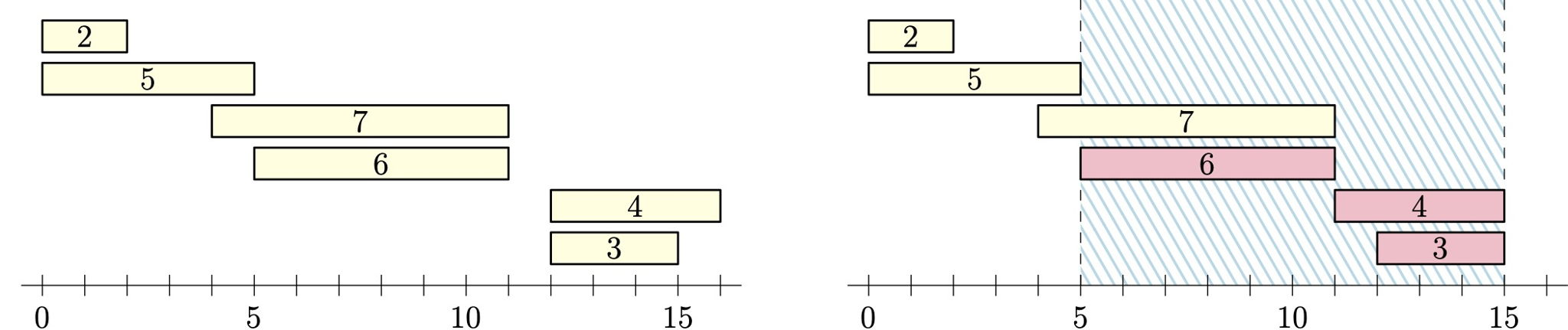시간 제한메모리 제한제출정답맞힌 사람정답 비율
3.5 초 (추가 시간 없음) 1024 MB0000.000%

## 문제

Alice wants to send $n$ messages to Bob over a communication channel. The $i$th message takes $t_i$ time steps to send.  At each integer time step, Alice can start sending any number of her messages.  Once started, a message must be transmitted in its entirety (it cannot be paused and resumed later).  Any number of messages can be sent in parallel over the channel without affecting the transmission time of individual messages.

An attacker has the capability to disable the security protocols of the channel for an interval of $x$ continuous time steps, but only once (i.e., after doing this, they cannot wait a while and then disable it for another $x$ time steps).  While the security is disabled, the attacker is able to listen in, and any message that is sent in its entirety during those $x$ time steps is considered exposed.

What is the minimum time needed for Alice to send all $n$ messages to Bob so that at most two messages are exposed, no matter when the attacker chooses to disable the security?Figure E.1: Left: Illustration of a solution to Sample Input 1.  Right: sending the message of length $4$ a time step earlier would not be a solution, because the three messages of length $6$, $4$, and $3$ would then be exposed to an eavesdropper listening in from time step $5$ to time step $15$.

## 입력

The first line of input contains the two integers $n$ and $x$ ($1 \leq n \leq 20\,000$, $1 \leq x \leq 10\,000$), the number of messages Alice wants to send and the number of time steps someone may listen in.  This is followed by a line containing $n$ integers $t_1, \ldots, t_n$ ($1 \leq t_i \leq 10\,000$), the number of time steps it takes to transmit each message.

## 출력

Output the minimum number of time steps to complete transmission of all $n$ messages so that at most two of them can be exposed.

## 예제 입력 1

6 10
2 3 4 5 6 7


## 예제 출력 1

16


## 예제 입력 2

7 6
9 3 2 3 8 3 3


## 예제 출력 2

11


## 출처• 문제를 만든 사람: Jimmy Mårdell